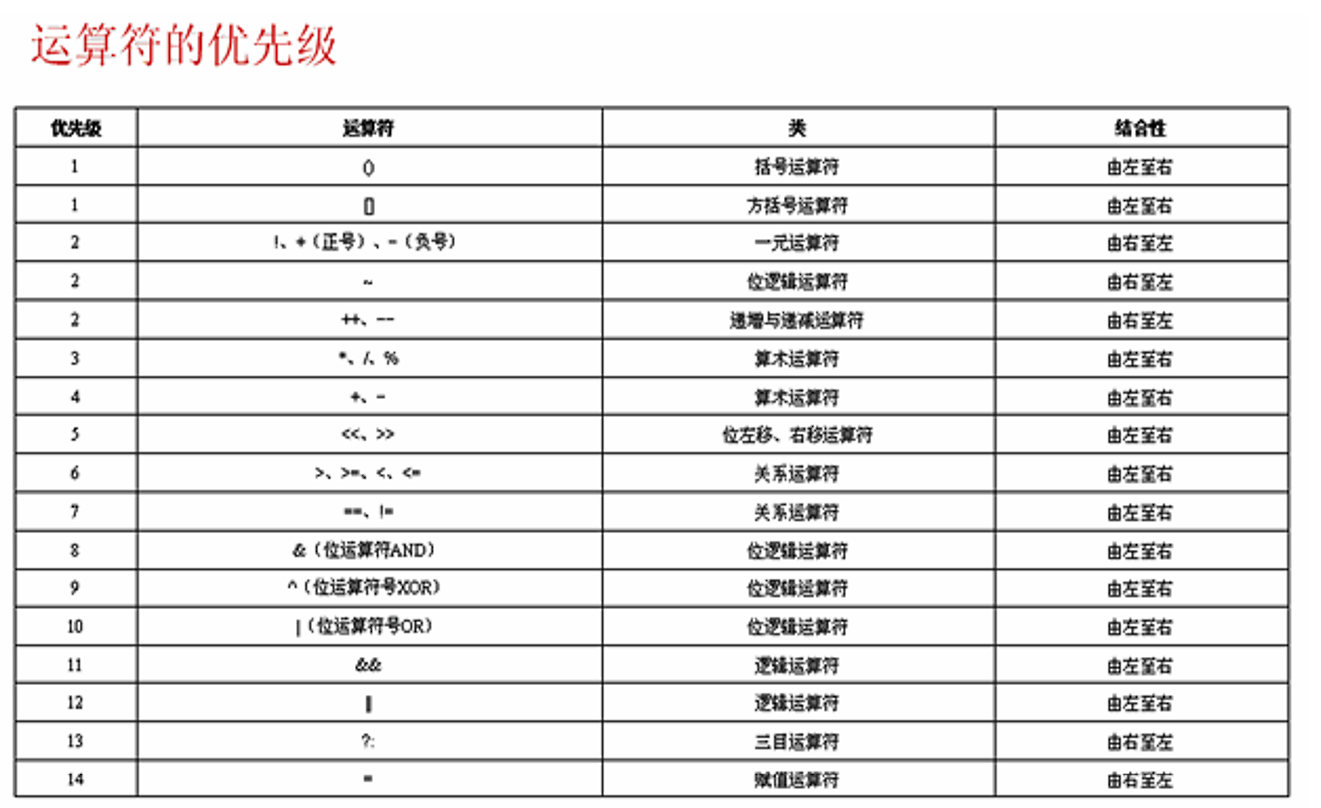# Java学习笔记-运算符与表达式

2020-12-19
Ko_teiru

Java学习笔记02

## 运算符与表达式

### 运算符

System.out.println(z+100);
// z与100都是操作数 而+就是运算符


#### 赋值运算符

= 赋值

String cloud="sb";


#### 一元运算符

+ 正号
- 符号
! NOT，否

boolean b=false;
int x=10;
int y=-30;
System.out.println("b="+b+", !b="+ !b); //取反
System.out.println("x="+x+", -x"+ -x);  //使用负号
System.out.println("y="+y+", +y"+ +y);  //使用正号


#### 算术运算符

+ 加法
- 减法
* 乘法
/ 除法
% 取模(求余数)

int i=10;
int j=3;
System.out.println(i+"+"+j+"="+(i+j));  //加法操作  10+3=13
System.out.println(i+"-"+j+"="+(i-j));  //减法操作  10-3=7
System.out.println(i+"*"+j+"="+(i*j));  //乘法操作  10*3=30
System.out.println(i+"/"+j+"="+(i/j));  //除法操作  10/3=3
System.out.println(i+"%"+j+"="+(i%j));  //取模操作  10%3=1


#### 自增自减运算符

++ 自增，变量值加1
-- 自减，变量值减1

int a=3,b=3;
int x=6,y=6;
System.out.println("a="+a);    //a=3
System.out.println("a++ ="+(a++)+",a ="+a);  //先计算后自增   a++=3,a=4
System.out.println("b ="+b);   //b=3
System.out.println("++b ="+(++b)+",b ="+b);  //先自增后计算   ++b=4,b=4
System.out.println("x ="+x);   //x=6
System.out.println("x-- ="+(x--)+",x ="+x);  //先计算后自增   x--6,x=5
System.out.println("y ="+y);   //y=6
System.out.println("--y ="+(--y)+",y ="+y);  //先自增后计算   --y=5,y=5


#### 逻辑运算符

& AND,与
&& 短路与
| OR,或
|| 短路或

• & 与：
• 要求前后几个表达式的内容都要是true，最终结果才会是true。如果有一个是false,那最终结果就是false
• 所有为真才为真，一假全假
• && 短路与
• 读取到一个假立马输出假，后续不进行判断
• | 或：
• 要求前后几个表达式只有一个为true则最终结果就是true。如果全部是false，最终结果才为false
• 所有为假才为假，一真全真
• || 短路或
• 读取到一个真立即输出一个真，后续不进行判断

if((23==23)||(100/0==0)){     //计算100/0会报错
System.out.println("真");
}else{
System.out.println("假");
}


if((23==32)&&(100/0==0)){
System.out.println("真");
}else{
System.out.println("假");
}


int x,y=10;   //这里是声明变量x为int形，而不是给x和y都赋值为10 还请注意
if(((x=0)==0)|| ((y=20)==20)){
System.out.println("现在y的值为："+y);      //现在y的值为：10
}
int a,b=10;   //a为int形，10赋予b
if(((a=0)==0)|((b=20)==20)){
System.out.println("现在b的值为："+b);      //现在b的值为：20
}


() 提高括号中运算符的优先级

:——-:|:–: &|按位与 | |按位或 ^ | 异或 ~ | 取反 <<|左位移 >>|右位移 >>>|无符号右位移

int a=3,b=5;
c=a>b?a:b;  //如果a>b满足，则输出结果1（a），如果不满足则输出b  c为5
System.out.println(a<c?a:c);  //输出结果为3+=：左右两个的内容相加之后再赋予左边的变量，如：

int a=3,b=3;
a+=b;   //先计算a+b，再将结果赋予a
System.out.println(a);  //输出6


int a=12,b=3;
a*=b++;   //先进行进行a*b，把结果赋予a。再进行b的自增，b+1
System.out.println("a="+a+",b="+b);   //a=36，b=4
int x=12,y=3;
x*=++y;   //先进行y的自增，y+1。再计算x*y，将结果赋予x
System.out.println("x="+x+",y="+y);   //x=48，y=4


### 单元小结

1. 清楚掌握JAVA中的赋值、计算、关系运算符
2. 了解一下位的运算（完全不了解）
3. 使用括号可以轻易的改变运算的优先级